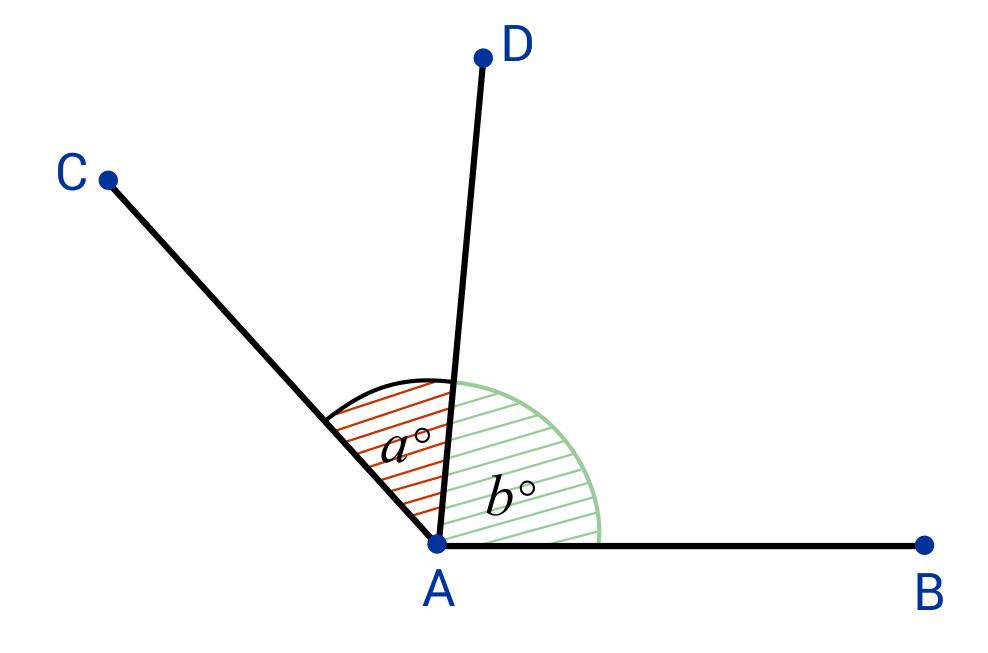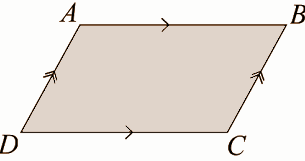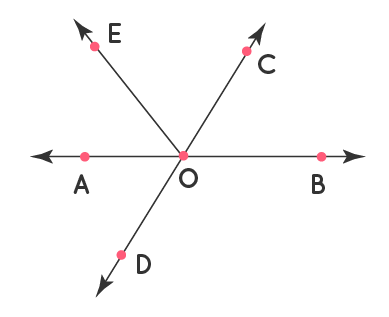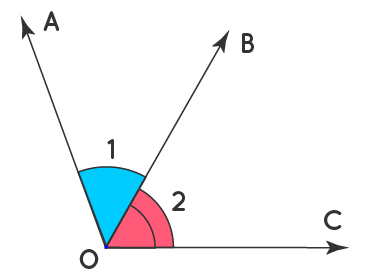Thursday, 18 Aug 2022

# Adjacent angle — all the explanations

Adjacent angles are two angles that share a vertex and a side. Moreover, the vertex of an angle is the point at which the rays that form the angle’s sides meet. When we say a common vertex and a common side, we mean that the two angles share the vertex point and the side. When we rotate a ray about its endpoint, the angle formed by the ray between its initial and final positions is known as the angle formed by the ray.

We use the pair of angles in geometry at times. Thus, there are several types of angle pairs, such as supplementary angles, complementary angles, adjacent angles, linear pairs of angles, opposite angles, and so on. In this article, we will go over the definitions of adjacent angles and vertical angles in great detail.When two angles share a common vertex and side, they are said to be adjacent angles. The vertex of an angle is defined as the endpoints of the ray from the side of an angle. When two adjacent angles share a common vertex and side, they can be complementary or supplementary angles.The two angles ∠BAC and ∠CAD in the diagram above have a common side (the blue line segment AC). They also share a vertex (point A). As a result, they are known as ‘adjacent angles.’ The larger angle ∠BAD is obviously the sum of the two adjacent angles.

180° is the sum of two angles.

The two angles will shift so that they always add up to 180 degrees.The two angles ∠PQR and ∠JKL in the diagram above are supplementary because they always add up to 180°.

Angle pairs are two angles that we join together. These angles’ measurements can sometimes form a special relationship. We know such one type of special relationship as complementary angles. Angle pairs that add up to exactly 90 degrees are known as complementary angles. In other words, when we combine the two angles, they form a right angle.They have the same vertex and common side.Angles 1 and 3 in the diagram are not adjacent.

They have a shared vertex but not a shared side.Angles 1 and 2 are not congruent.

They have a shared side but not a shared vertex.

If you find The sum of supplementary adjacent angles, then you’ll find is always 180 degrees. This is due to the fact that the two angles are adjacent on a straight line, and all rremaining angles on a straight line add up to 180. Again, if the adjacent angles are not linear pairs and there is another angle in the mix, the two adjacent angles will not add up to 180.

There are numerous real-world examples of adjacent angles.

• The most common real-world example of adjacent angles is two pizza slices placed next to each other.
• Another common example is a clock, which displays the hour, minute, and second hands, which form adjacent angles when all three are separated.
• In the steering wheel of a car, we can find three adjacent angles.

The properties of adjacent angles are listed below to assist us in identifying them.

• Adjacent angles are always connected by a common arm.
• They are connected by a vertex.
• They do not intersect.
• On both sides of the common arm, they have a non-common arm.
• Based on the sum of the individual angles’ measures, two adjacent angles can be supplementary or complementary.

## How to identify adjacent angles

Adjacent angles are easily distinguished by two key characteristics: they always have a common side and a common vertex. If two angles satisfy only one of these criteria, they are not considered adjacent. It is necessary for the angles to satisfy both properties. For example, if two angles share a common vertex but are separated by an angle, this indicates that they do not share a common side. As a result, they cannot be adjacent angles. To identify adjacent angles, look at the figure below.

## What is the difference between a vertical and an adjacent angle?

Identifying the difference between adjacent and vertical angles is a crucial skill in geometry. To realise the difference between these two types of angles, imagine two straight lines intersecting to form a cross.

Four angles are formed when a cross is formed. We can recognise adjacent angles because they share a common side and a common vertex. But how do we know what a vertical angle is? Finding a vertical angle is as simple as finding an adjacent angle. A set of vertical angles, like adjacent angles, will have a vertex point in common. They do not, however, have to share a common side.

When considering a cross, the vertical angles are those that are opposite each other. As a result, they are sometimes referred to as vertically opposite angles.

The simplest way to identify an adjacent angle is to be able to identify a common side and a common vertex. When two angles share one side and derive from the same corner (vertex), they are said to be adjacent angles.

It’s critical to remember that adjacent angles must share a common vertex as well as a common side. As a result, if you see two angles coming from the same corner but another angle in the middle, it means they don’t share any sides. Because they do not share a side AND a vertex, they are not adjacent angles.

• They have a common side
• They have a common vertex
• The angles do not overlap
• Although they share a common side in the centre, no other sides are shared
• No common interior point
• They can be complementary or supplementary

## Adjacent angle of a parallelogram

The parallelogram’s adjacent angles are also known as the parallelogram’s adjacent angles that are adjacent to each other. A parallelogram has four pairs of adjacent angles that are supplementary.

Adjacent angles of a parallelogram are those that we place next to each other in order. Look at the parallelogram below to see the four pairs of adjacent angles. We also know these angles as parallelogram consecutive angles. Each adjacent angle here adds up to 180°. The parallelogram has four pairs of adjacent angles: ∠A and ∠B, ∠B and ∠C, ∠C and ∠D, and ∠D and ∠A.## Properties of the Adjacent Angles of a Parallelogram

The properties of a parallelogram’s adjacent angles are as follows:

• A parallelogram’s adjacent angles are supplementary.
• A parallelogram has four pairs of adjacent angles.
• If the adjacent angles of a parallelogram are equal, the parallelogram is a rectangle; if the sides are equal, the parallelogram is a square.

• When two angles are adjacent, the angle formed by two non-common arms and one common arm is the sum of the two angles.
• If a ray follows a straight line, the sum of the adjacent angles formed is 180°.
• A linear pair of angles are formed when the sum of two adjacent angles is 180°. Because supplementary angles add up to 180°, all linear pairs are supplementary. All supplementary angles, however, do not have to be linear pairs. Lines must intersect and form adjacent angles in order to form a linear pair.
• The non-common arms form a line if the sum of two adjacent angles is 180°.

## Two Adjacent Angles of a Parallelogram are Supplementary

A parallelogram’s two adjacent angles are always supplementary. This means that the sum of these consecutive angles is 180°. Let us examine this fact with the help of the following evidence. Consider the following diagram in relation to the proof given below.

Proof:

If ABCD is a parallelogram, we know the opposite sides are parallel.

• Let us take AB || DC where AD and BC become the transversals.
• When a transversal cuts two parallel lines, the co-interior angles on the same side of the transversal are supplementary. Therefore, here,
• ∠A + ∠D = 180°, also ∠B + ∠C = 180°
• Now, if AD || BC, then the lines AB and DC become transversals. We know the co-interior angles in two parallel lines are supplementary. Thus ∠A + ∠B = 180°, and ∠D + ∠C = 180°
• Therefore, the sum of any two adjacent angles is supplementary. Which means, ∠A + ∠B = 180°, ∠B + ∠C = 180°, ∠C + ∠D = 180°, and ∠D + ∠A = 180°.## Adjacent angle of a trapezium

We refer to the two angles on the same leg of a trapezoid (one on the top base, one on the bottom base) as ‘adjacent angles.’ These adjacent angles are supplementary, which means that their measurements add up to 180°, as we will see later.

## Adjacent angle of a rhombus

The interior angles of a rhombus are four. The sum of a rhombus’ interior angles equals 360 degrees. So, a rhombus’s opposite angles are equal to each other. Hence, the adjacent angles are extraneous.

If two quadrilateral angles share a side as an arm, then we call them adjacent angles. There are four adjacent angles

### Vertically opposite angles

Vertically opposite angles are not technically adjacent angles, but where there are adjacent angles, there are likely to be some vertically opposite angles as well.

We have already investigated the vertical angles, but to clarify, vertical angles share the same vertex but no sides. In the example above, we consider the angles 3 and 4 and 1 and 2 to be vertically opposite.

One distinguishing feature of vertically opposite angles is that they have the same measurement. For example, if angle 1 is 30 degrees, angle 2 is also 30 degrees.

We have given below a few adjacent angles examples.

Example 1: Find all the pairs of adjacent angles in the given figure.Solution: There are five pairs of adjacent angles. We have mentioned them below.

∠AOE, ∠EOC

∠EOC, ∠COB

∠COB, ∠BOD

∠BOD, ∠AOD

∠AOD, ∠AOE

Example 2: Mention if the angles marked as 1 and 2 in the following figures are adjacent? Give reasons for your answers.

Solution: Clearly ∠1, ∠2 have a common vertex O and a common ray OB. Therefore, ∠1, ∠2 are adjacent angles.## Difference between adjacent angle and vertical angle

• Adjacent angles are two angles that share a common arm and vertex. When two lines intersect, the pair of opposite angles we see at the vertex is referred to as a vertical angle.
• Adjacent angles have a common arm and vertex. Vertical angles have a shared vertex but no shared arm.
• Adjacent angles are not always the same size. Vertical angles that are vertically opposite are equal in size.

### 1. What exactly are Adjacent Angles?

Simply put, adjacent angles are angles with a common side and a common vertex.

### 2. Is adjacent angle equal to 180 degrees?

Angles A and B are adjacent. The sum of adjacent angles is 180 degrees. These sum to 180 degrees (E And C Are Also Interiors). Supplementary Angles are any two angles that add up to 180 degrees.

Sides that are adjacent. Two lines intersect at a polygon vertex. Adjacent Sides are two sides that meet at a vertex of a polygon and we commonly find them in triangles and other polygons.

### 4. Can two obtuse angles be adjacent?

Is it possible for two obtuse angles to be adjacent? Yes. It is possible for two obtuse angles to be adjacent. It is, in other words, between a right angle and a straight angle.

### 5. Is it true that adjacent angles are always equal?

Vertical angles are always congruent, which means they are all the same. Adjacent angles are those that emerge from the same vertex. Furthermore, a common ray connects the adjacent angles and they do not overlap.

### 6. Is it possible for two adjacent angles to be complementary?

Adjacent angles are two angles that share a common vertex, a common side, but no interior points. The Angles Are Supplementary If Two Angles Form A Linear Pair. A Linear Pair creates a straight angle that contains 180o, so you have two angles whose measurements add up to 180, indicating that they are complementary.

### 7. Is it possible for two adjacent angles to be complementary?

Two adjacent angles that add up to 90° can also be complementary. The altitude from a right-angled vertex in a right triangle will split the right angle into two adjacent angles; 30°+60°, 40°+50°, and so on. Those Adjacent Angles Complement Each Other. Two adjacent angles can also be supplemental if they add up to 180°.

### 8.  Is it possible for adjacent angles to be linear pairs?

YES! Adjacent angles can indeed form linear pairs. Because linear pairs have a common side as well as a common vertex, they can be considered adjacent angles. Not all adjacent angles, however, are linear pairs.

### 9.  Can Adjacent Vertical Angles Exist?

Because vertical angles and adjacent angles are frequently found in close proximity, we all believe that vertical angles are indeed adjacent angles. This is a FALSE statement. Vertical angles do not have any sides in common, so they cannot be adjacent.

### 10. Are adjacent angles equal?

Vertical angles are congruent, this means that they are equal. Adjacent angles are angles that come out of the same vertex. Therefore, adjacent angles share a common ray and do not overlap.

### 11.Is it true that vertical angles are always complementary?

Vertical angles are all the same size. As a result, if two vertical angles are 45o apart, they are complementary.